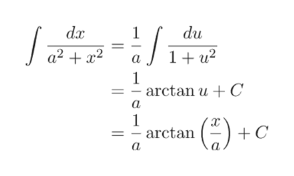Question
1 views

Find or evaluate the integral by completing the square. (Use C for the constant of integration.)

check_circle

star
star
star
star
star
1 Rating
Step 1

Let's look at the denominator.

The denominator = 9x2 – 18x + 36 = 9x2 – 18x + 9 + 27 = (9x2 – 18x + 9) + 27 = 9[(x2 – 2x + 1) + 3] = 9[(x – 1)2 + 3]

Step 2

Please see the white board. Let x - 1 = z; hence, dx = dz

The integral therefore takes the shape as shown in the last expression on the white board.

Step 3

Recall the standard integration ...help_outlineImage Transcriptionclosedar du a2+ x2 1+ u2 a 1 arctan uC 1 arctan C = fullscreen

### Want to see the full answer?

See Solution

#### Want to see this answer and more?

Solutions are written by subject experts who are available 24/7. Questions are typically answered within 1 hour.*

See Solution
*Response times may vary by subject and question.
Tagged in

### Calculus